# Finding Intercepts of Rational Fractions

Intercepts are the points at which a graph crosses either the x or y axis, and they are very useful in sketching functions.

To find the y-intercept(s) (the point where the graph crosses the y-axis), substitute in 0 for x and solve for y or f(x).

To find the x-intercept(s) (the point where the graph crosses the x-axis â also known as zeros), substitute in 0 for y and solve for x.

Examples: Find the intercepts of the function given.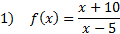To find the y-intercept, we must substitute in 0 for each x:And then simplify: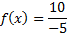There is a y-intercept at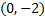. (Notice that 0 is the x coordinate because on the y-axis, x = 0.)

To find the x-intercept, we must substitute in 0 for y or f(x):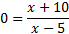And then solve by cross-multiplying: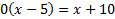0 = x + 10

x = -10

There is a y-intercept at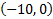. (Notice that 0 is the y coordinate because on the x-axis, y = 0.)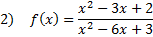To find the y-intercept, we must substitute in 0 for each x: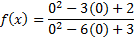And then simplify:There is a y-intercept at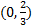.

To find the x-intercept, we must substitute in 0 for y or f(x):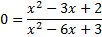And then solve by cross-multiplying: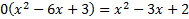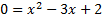We must now solve the quadratic either by factoring or by using the quadratic formula.
We can factor this trinomial, so we'll use that method:There are y-intercepts at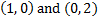.

Note: Not all rational functions have both an x or y intercept. If you cannot find a real solution, then it does not have that intercept.

Practice: Find the x and y intercepts of each rational function: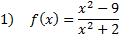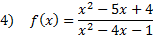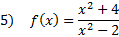Answers: 1)x-int.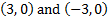y-int.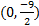2) x-int. (4, 0) y-int.3) x-int. (-2, 0) and (5, 0) y-int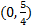4) x-int. (1, 0) and (4, 0) y-int (0, -4) 5) x-int: none y-int: (0, -2)